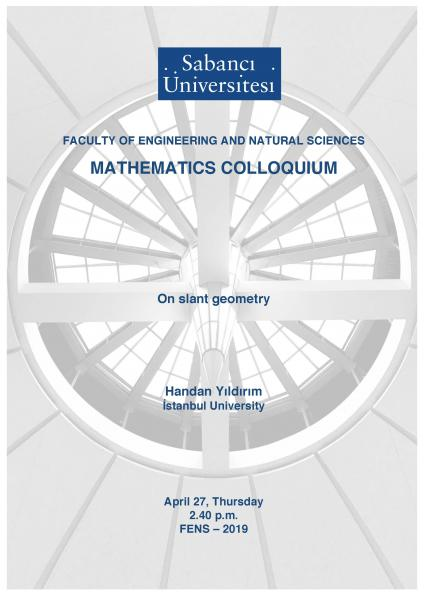## Mathematics Colloquium: On Slant Geometry

### Mathematics Colloquium: On Slant GeometrySpeaker: Handan Yildirim (Istanbul University)

Title: On Slant Geometry

Date/Time: Thursday, April 27, 2017 14:40 - 15:30

Place: FENS G029

Abstract: It is known that a geodesic in the Poincar ́e disk is a circle which is perpendicular to the ideal boundary (i.e., unit circle). If we adopt geodesics as lines in the Poincar ́e disk, we have the model of Hyperbolic geometry. We have another class of curves in the Poincar ́e disk which has an analogous property with lines in the Euclidean plane. A horocycle is a circle which is tangent to the ideal boundary. We note that a line in the Euclidean plane can be considered as the limit of the circles when the radius tends to infinity. In the same manner, a horocycle is also a curve which can be considered as the limit of the circles in the Poincar ́e disk when the radius tends to infinity. Hence, horocycles are also an analogous notion of lines. If we adopt horocycles as lines, what kind of geometry do we obtain? We say that two horocycles are parallel if they have common tangent point at the ideal boundary. Under this definition, the parallel axiom is satisfied. However, for any two fixed points in the Poincar ́e disk, there exist always two horocycles passing through these points, so that the first axiom of Euclidean geometry is not satisfied. In the case of general dimensions, this geometry is said to be horospherical geometry. On the other hand, we have another kind of curves in the Poincar ́e disk which has similar properties with Euclidean lines. An equidistant curve is a circle whose intersection with the ideal boundary consists of two points. Generally, the angle between an equidistant curve and the ideal boundary is φ ∈ (0, π/2]. Here, we emphasize that a geodesic is an equidistant curve with φ = π/2. However, a horocycle is not an equidistant curve, but it is a circle with φ = 0. We call the geometry where φ = π/2 vertical geometry and the geometry where φ = 0 horizontal geometry. And also we call the family of geometry depending on φ slant geometry.

Contact: Yasemin Şengül